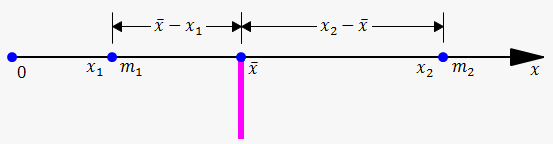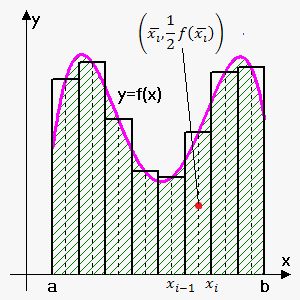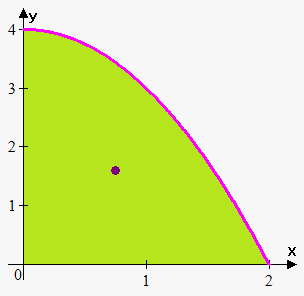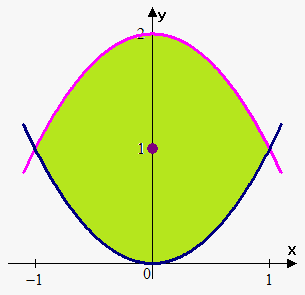# Moments and Centers of Mass

In this note we will try to find a point P on which a thin plate of any given shape balances horizontally. This point is called the center of mass (or center of gravity) of the plate.

We first consider the simpler situation. Suppose that the rod lies along the x-axis with m_1 at x_1 and m_2 at x_2 and the center of mass at barx. According to the Law of Lever We seem_1(barx-x_1)=m_2(x_2-barx) or barx=(m_1x_1+m_2x_2)/(m_1+m_2).

The numbers m_1x_1 and m_2x_2 are called the moments of the masses m_1 and m_2 (with respect to the origin). Above equation says that centers of mass is obtained by adding the moments of masses and dividing by total mass.

In general, if we have a system of n particles with masses m_1,m_2,...,m_n located at the points x_1,x_2,...,x_n on the x-axis, it can be shown similarly that the center of mass of the system is located at barx=(sum_(i=1)^nm_ix_i)/(sum_(i=1)^n m_i).

Sum of the individual moments M=sum_(i=1)^n m_ix_i is called the moment of the system with respect to the origin and m=sum_(i=1)^nm_i is the total mass of the system.

Now we consider a system of particles with masses m_1,m_2,...,m_n located at the points (x_1,y_1),(x_2,y_2),...,(x_n,y_n) in the xy-plane. By analogy with the one-dimensional case, we define the moment of the system about the y-axis to be M_y=sum_(i=1)^nm_ix_i and the moment of the system about x-axis as M_x=sum_(i=1)^n m_iy_i.

M_y measures the tendency of the system to rotate about the y-axis and M_x measures the tendency to rotate about the x-axis.
As in the one-dimensional case, the coordinates of the center of mass are given in terms of the moments by the formulas barx=(M_y)/m and bary=(M_x)/m where m=sum_(i=1)^nm_i is the total mass. Since m barx=M_y and m bary=M_x, the center of mass (barx,bary) is the point where a single particle of mass m would have the same moments as the system.

Example 1. Find the moments and center of mass of the system of objects that have masses 5, 7, and 8 at the points (2,1), (3,-5), and (-4,4).

M_y=5*2+7*3+8*(-4)=-1 .

M_x=5*1+7*(-5)+8*4=2 .

Since m=5+7+8=20 then barx=(M_y)/m=-1/20 and bary=(M_x)/m=2/20=1/10.

So, center of mass is located at point (-1/20,1/10).Next we consider a flat plate (called a lamina) with uniform density rho that occupies a region R under continuous curve y=f(x), above x-axis and between lines x=a and x=b. We
wish to find the center of mass of the plate, which is called the centroid of R. In doing this we use the following simple fact: centroid of a rectangle is its center.

As always we divide the interval [a,b] into n subintervals with endpoints x_0,x_1,...,x_n and equal width Delta x. We choose the sample point x_i^(**) to be the midpoint bar(x_i) of the i-th subinterval, that is, bar(x_i)=1/2(x_(i-1)+x_i).

The centroid of the i-th approximating rectangle R_i is its center C_i=(bar(x_i),1/2f(bar(x_i))). Its area is f(bar(x_i))Delta x, so its mass is rho f(bar(x_i))Delta x.

The moment of R_i about the y-axis is the product of its mass and the distance from C_i to the y-axis, which is bar(x_i). Thus, M_y(R_i)=(rho f(bar(x_i))Delta x)bar(x_i)=rho bar(x_i)f(bar(x_i))Delta x. Adding these moments, we obtain the moment of the polygonal approximation to R, and then by taking the limit as n->oo we obtain the moment of R itself about the y-axis: M_y=lim_(n->oo)sum_(i=1)^n rho bar(x_i)f(bar(x_i))Delta x=rho int_a^bxf(x)dx.

Similarly we compute the moment of R_i about the x-axis as the product of its mass and the distance from C_i to the x-axis: M_x(R_i)=(rho f(bar(x_i))Delta x)1/2f(bar(x_i))=1/2rho (f(bar(x_i)))^2Delta x.
Again we add these moments and take the limit to obtain the moment of R about the x-axis:

M_x=lim_(n->oo)sum_(i=1)^n 1/2 rho(f(bar(x_i)))^2Delta x=rho int_a^b 1/2 (f(x))^2dx.

Just as for systems of particles, the center of mass of the plate is defined so that mbar(x)=M_y and mbar(y)=M_x. But the mass of the plate is the product of its density and its area: m=rho A=rho int_a^b f(x)dx.

Therefore,

bar(x)=(M_y)/m=(rho int_a^b xf(x)dx)/(rho int_a^b f(x)dx)=(int_a^b xf(x)dx)/(int_a^b f(x)dx) and bar(y)=(M_x)/m=(rho int_a^b 1/2(f(x))^2dx)/(rho int_a^b f(x)dx)=(int_a^b 1/2 (f(x))^2dx)/(int_a^b f(x)dx).

Note, that center of mass doesn't depend on density.

The center of mass of the plate (or the centroid of R) is located at the point (bar(x),bar(y)), where

barx=1/A int_a^b xf(x)dx =(int_a^b xf(x)dx)/(int_a^b f(x)dx)

bary=1/A int_a^b 1/2 (f(x))^2dx=(int_a^b 1/2 (f(x))^2dx)/(int_a^b f(x)dx).

Example 2. Find the center of mass of region bounded by y=4-x^2 for x>=0.First let's find area: A=int_0^2(4-x^2)dx=(4x-1/3 x^3)|_0^2=4*2-1/3 *8=16/3.

Now, bar(y)=1/A int_0^2 1/2 (4-x^2)^2dx=3/(32) int_0^2(16-8x^2+x^4)dx=

=3/32 (16x-8/3 x^3+1/5 x^5)|_0^2=3/32 *256/15=8/5.

bar(x)=1/A int_0^2 x(4-x^2)dx=3/(16) int_0^2(4x-x^3)dx=

=3/16 (2x^2-1/4 x^4)|_0^2=3/16 *4=3/4.

So, center of mass is (3/4,8/5).

In a similar way if region R is bounded by curves f(x) and g(x) on interval [a,b] and f(x)>=g(x) for a<=x<=b then

The center of mass of the plate (or the centroid of R) is located at the point (bar(x),bar(y)), where

barx=1/A int_a^b x(f(x)-g(x))dx =(int_a^b x(f(x)-g(x))dx)/(int_a^b (f(x)-g(x))dx)

bary=1/A int_a^b 1/2 ((f(x))^2-(g(x))^2)dx=(int_a^b 1/2 ((f(x))^2-(g(x))^2)dx)/(int_a^b (f(x)-g(x))dx).

Example 3. Find center of mass of the region bounded by y=x^2 and y=2-x^2.First we sketch graph and see that bounds of integration are -1 and 1.

Next, since region is symmetric about x-axis then bar(x)=0.

A=int_(-1)^1 (2-x^2-x^2)dx=(2x-2/3 x^3)|_(-1)^1=8/3.

bar(y)=1/A int_(-1)^1 1/2 ((2-x^2)^2-(x^2)^2)dx=3/16 int_(-1)^1 (4-4x^2)dx=

=3/16 (4x-1/3 x^3)|_(-1)^1=3/16* 16/3.

So, center of mass is (0,1). Alternatively we could notice that region is symmetric about line y=1, so bar(y)=1.